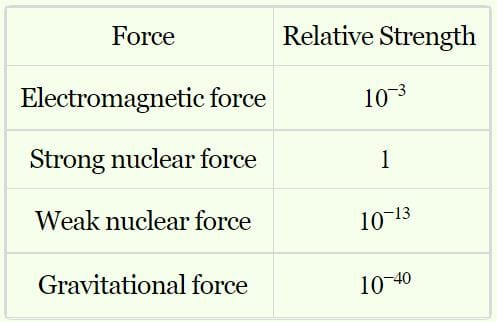NEET  >  Test: Fundamental Forces In Nature

# Test: Fundamental Forces In Nature

Test Description

## 10 Questions MCQ Test Physics Class 11 | Test: Fundamental Forces In Nature

Test: Fundamental Forces In Nature for NEET 2023 is part of Physics Class 11 preparation. The Test: Fundamental Forces In Nature questions and answers have been prepared according to the NEET exam syllabus.The Test: Fundamental Forces In Nature MCQs are made for NEET 2023 Exam. Find important definitions, questions, notes, meanings, examples, exercises, MCQs and online tests for Test: Fundamental Forces In Nature below.
Solutions of Test: Fundamental Forces In Nature questions in English are available as part of our Physics Class 11 for NEET & Test: Fundamental Forces In Nature solutions in Hindi for Physics Class 11 course. Download more important topics, notes, lectures and mock test series for NEET Exam by signing up for free. Attempt Test: Fundamental Forces In Nature | 10 questions in 10 minutes | Mock test for NEET preparation | Free important questions MCQ to study Physics Class 11 for NEET Exam | Download free PDF with solutions
 1 Crore+ students have signed up on EduRev. Have you?
Test: Fundamental Forces In Nature - Question 1

### Earth pulls every object towards it with a force called:

Detailed Solution for Test: Fundamental Forces In Nature - Question 1

Gravitational force of the earth acts from the centre of the earth and pulls every substance towards itself.Test: Fundamental Forces In Nature - Question 2

### What is the study of the modern theory of the strong forces between quarks?

Detailed Solution for Test: Fundamental Forces In Nature - Question 2

Quantum chromodynamics is as quantum field theory in which strong interaction is described in terms of an interaction between quarks.

Test: Fundamental Forces In Nature - Question 3

### Gravity is:

Detailed Solution for Test: Fundamental Forces In Nature - Question 3

Gravity acts from the centre of the earth, therefore it is a down ward acting force.

Test: Fundamental Forces In Nature - Question 4

The strongest fundamental force in nature is

Detailed Solution for Test: Fundamental Forces In Nature - Question 4Test: Fundamental Forces In Nature - Question 5

Gravity is defined  as:

Detailed Solution for Test: Fundamental Forces In Nature - Question 5

Gravitational force of the earth acts from the centre of the earth and pulls every substance towards itself.

Test: Fundamental Forces In Nature - Question 6

Which force is weakest of all fundamental forces?

Detailed Solution for Test: Fundamental Forces In Nature - Question 6

Gravitational force is the attraction between two masses.

Electromagnetic force is the force between two charged particles or two poles of a magnet. It is stronger than Gravitational.

Weak nuclear force is the weak force of attraction between the subatomic particles of the nucleus of an atom. Though the name suggests that it is weak, it is stronger than electromagnetic.

Strong nuclear force is the stronger version of nuclear force.It is the strongest force in nature.

Test: Fundamental Forces In Nature - Question 7

Earth has no gravitational force:

Detailed Solution for Test: Fundamental Forces In Nature - Question 7

Gravitational force of the earth acts from the centre of the earth and pulls every substance towards itself.

Test: Fundamental Forces In Nature - Question 8

Wheel-and-axle and rope move in:

Detailed Solution for Test: Fundamental Forces In Nature - Question 8

Wheel-arid-axle and rope move in same direction.

Test: Fundamental Forces In Nature - Question 9

Which of these is incorrect about nuclear forces?

Detailed Solution for Test: Fundamental Forces In Nature - Question 9

Hadrons are subatomic composite porticle made of two(or) more quarks. They experience strong nuclear force. ​

Test: Fundamental Forces In Nature - Question 10

Which of the following class of forces is different from others?

Detailed Solution for Test: Fundamental Forces In Nature - Question 10

Spring force is different from magnetic ,electrical and gravitational force as it varies linearly.

## Physics Class 11

124 videos|464 docs|210 tests
Information about Test: Fundamental Forces In Nature Page
In this test you can find the Exam questions for Test: Fundamental Forces In Nature solved & explained in the simplest way possible. Besides giving Questions and answers for Test: Fundamental Forces In Nature, EduRev gives you an ample number of Online tests for practice

## Physics Class 11

124 videos|464 docs|210 tests

### How to Prepare for NEET

Read our guide to prepare for NEET which is created by Toppers & the best Teachers(Scan QR code)